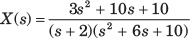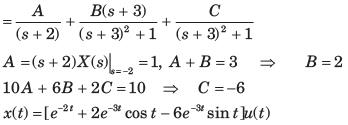Courses

# Test: Laplace Transform- 2

## 10 Questions MCQ Test Signals and Systems | Test: Laplace Transform- 2

Description
This mock test of Test: Laplace Transform- 2 for Electrical Engineering (EE) helps you for every Electrical Engineering (EE) entrance exam. This contains 10 Multiple Choice Questions for Electrical Engineering (EE) Test: Laplace Transform- 2 (mcq) to study with solutions a complete question bank. The solved questions answers in this Test: Laplace Transform- 2 quiz give you a good mix of easy questions and tough questions. Electrical Engineering (EE) students definitely take this Test: Laplace Transform- 2 exercise for a better result in the exam. You can find other Test: Laplace Transform- 2 extra questions, long questions & short questions for Electrical Engineering (EE) on EduRev as well by searching above.
QUESTION: 1

### Determine the Laplace transform of given signal. Q.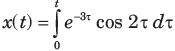Solution: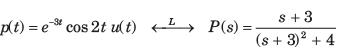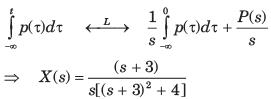QUESTION: 2

### Determine the Laplace transform of given signal. Q.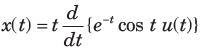Solution: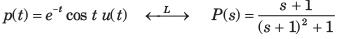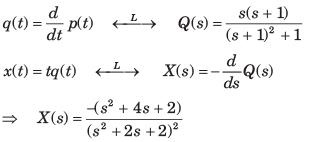QUESTION: 3

### Determine the time signal x(t) corresponding to given X (s) and choose correct option. Q.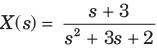Solution: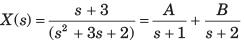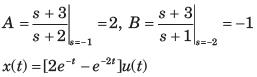QUESTION: 4

Determine the time signal x(t) corresponding to given X (s) and choose correct option.
Q.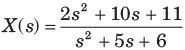Solution: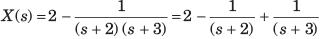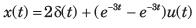QUESTION: 5

Determine the time signal x(t) corresponding to given X (s) and choose correct option.
Q.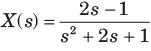Solution: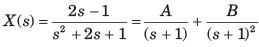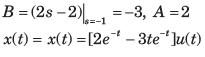QUESTION: 6

Determine the time signal x(t) corresponding to given X (s) and choose correct option.
Q.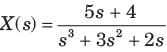Solution: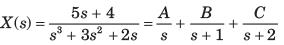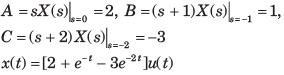QUESTION: 7

Determine the time signal x(t) corresponding to given X (s) and choose correct option.
Q.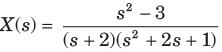Solution: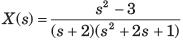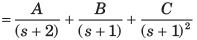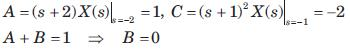x(t) = ( e-t - 2te-2t) u(t)

QUESTION: 8

Determine the time signal x(t) corresponding to given X (s) and choose correct option.
Q.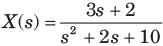Solution: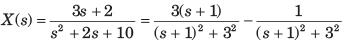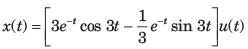QUESTION: 9

Determine the time signal x(t) corresponding to given X (s) and choose correct option.
Q.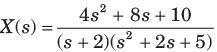Solution: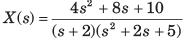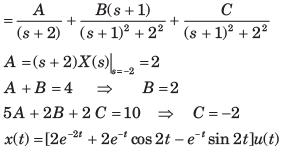QUESTION: 10

Determine the time signal x(t) corresponding to given X (s) and choose correct option.
Q.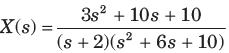Solution: